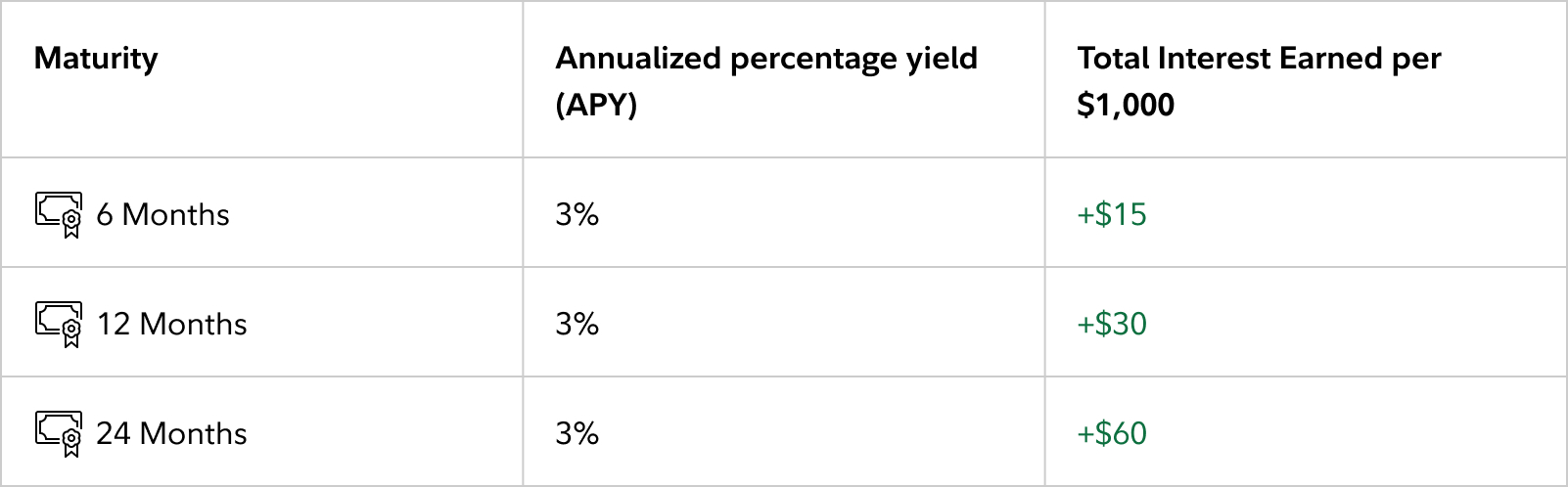## Understanding how your bond or CD earns money

Bonds and certificates of deposit (CDs) generally pay interest at predetermined intervals. Interest payments are commonly referred to as coupons. Sometimes, bonds can be issued as a ‘zero coupon’. In these cases, the bond is typically issued at a discount (meaning at a price less than par) and mature at par value. This article focuses on bonds and CDs with interest (or coupon) payments.

If you buy a bond or CD at par value, then the interest rate is the same as the annual percentage yield (APY), provided that you hold the CD through the maturity date and the security doesn’t default. APY is the amount you make on an investment, which is expressed as an annualized rate of return. All interest earned from bonds and CDs is paid to the core position as simple interest.

Let's look at a few examples using a new issue CD:

Let's say you invest \$1,000 into a CD paying 3% APY and that the CD matures in one year (365 days). At maturity, the interest you earn would be \$30 (3% of \$1,000 = \$30). Because the CD pays interest semiannually, you would be paid \$15 of interest after six months and another \$15 at maturity. When the CD matures, you would also receive the initial \$1,000 principal you invested.In our next example, you invest \$1,000 into a CD paying 3% APY but the CD matures in just six months (180 days). Although you are earning at the same rate of return, you are only investing for half as long. Therefore, the CD earns half as much as in the one-year example (a total of \$15 in interest). Remember, the total return you receive in cash terms is proportionate to the length of the investment period.In the final example, you invest \$1,000 into a CD paying 3% APY but the CD matures in two years (730 days), so the total interest you earn would be \$60. Because the CD pays semiannually, you would earn \$15 of interest after 6 months, 12 months, 18 months, and a final \$15 at the two-year maturity mark. As with the other examples, you would also receive the \$1,000 of principal that was initially invested when the CD matures.The total potential amount you can earn on a new issue CD investment is expressed as APY. With bonds and brokered CDs trading in the secondary market you will see the same concept of an annualized yield depicted as the Yield, specifically the “Yield to Maturity” or the “Yield to Worst”. The Yield to Worst is equal to the Yield to Maturity if the bond or CD is call protected, and for callable bonds it is the lower of either the Yield to Maturity and the Yield to Call. Remember, the APYs or yields displayed are annualized calculations and assume that the instrument is held through maturity or its call date.